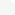# Categories# Graphics & Design → Mathworks Matlab R2017a 9.2.0.538062 Linux x64Mathworks Matlab R2017a 9.2.0.538062 Linux x64 | 9.63 GB

MATLAB (abbreviation for English "Matrix Laboratory") is a package of applied programs for solving technical computing tasks and the programming language of the same name used in this package. With the help of MATLAB created by MathWorks, you can easily perform matrix calculations, visualize mathematical functions and experimental data, implement computational algorithms, design a graphical user interface for solving specific problems, and interact with other programming languages ​​and programs via special interfaces.

# Search

«    March 2019    »
MonTueWedThuFriSatSun
123
45678910
11121314151617
18192021222324
25262728293031

» ReleaseLog
» Gfxtorrent